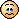Mis à jour le 04/10/2021

## Explore your data visually with Seaborn

Connectez-vous ou inscrivez-vous gratuitement pour bénéficier de toutes les fonctionnalités de ce cours !Seaborn  is a library that improves Matplotlib's functionality, replaces some default settings and functions, and adds new features.

Seaborn was created to correct three defects of Matplotlib. As a standalone, Matplotlib:

• Can't generate graphics of high aesthetic quality (especially in pre 2.0 versions).

• Lacks the functionality to easily create sophisticated statistical analyses.

• Features functions that aren't designed to interact with Panda Dataframes (which we will see in the next chapter).

Luckily, Seaborn addresses these problems! It still uses Matplotlib "under the hood", but does so by exposing more intuitive functions.

``````import seaborn as sns
sns.set()
x = np.linspace(0, 10, 500)
y = np.random.randn(500)
plt.plot(x,y)
``````

What do you think of the above graph? Do you find it more appealing to the eye?

Seaborn also provides us with functions to generate useful plots for statistical analysis. For example,`distplot` lets you not only view the histogram of a sample, but also estimate the distribution from which the sample is derived.

``````sns.distplot(y, kde=True);
``````

As I mentioned, Seaborn is really good at visualizing relationships and helping us draw insights from our data. What we will do now is demonstrate Seaborn's capacities using a very simple dataset called "Iris". This dataset is popular in introductory stats classes.

It contains 150 rows done on 3 different plant species. Each row is an observation of a certain species of plant. The observation contains quantitive columns including length and width of its sepals and petals.

``````iris = sns.load_dataset("iris")
``````

We can actually visualize the relationship between all these variables using a powerful function in Seaborn called `.pairplot`.

Conveniently, you only need one line of code to do this with Seaborn! We simply need to pass "Iris" as the data parameter, set the hue to species, and the size to 2.5.

``````sns.pairplot(iris, hue='species', height=2.5);
``````

You may be thinking: "What a nice visualization, but, how do I read this?"

Let me explain.

Basically, each variable (sepal_length, sepal_width, pedal_length, and pedal_width) is represented on both the X and Y axes.

Histograms occur when variables are crossed with themselves, for example, when sepal_length on the X axis is crossed with sepal_length on the Y axis. Of course, we have separated the data by species, whereby blue represents setosa, green represents versicolor, and red represents virginica.

The other elements are actually bivariate plots of the variables. For example, the first plot in the third column represents a bivariate plot of sepal_length and pedal_length. This type of output is really useful for drawing insights. For example, in this same plot, we can easily observe the linear relationship between sepal length and petal length!

We can also represent the joint distribution of two characteristics:

``````with sns.axes_style('white'):
sns.jointplot("petal_length", "petal_width", data=iris, kind='reg')
``````
• Use  `displot`  to estimate a graph's distribution.
• Visualize the relationship between variables using  `.pairplot`  .